/

### The hypergeometric distribution

The hypergeometric distribution describes the probability of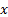successes indraws from a finite population size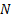containing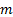successes without replacement. This contrasts with the binomial distribution which describes the probability ofsuccesses indraws with replacement

 Distribution name Hypergeometric distribution Common notation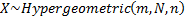Parameters= population size, integral (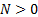)= sample size, integral (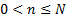)= number of tagged items, integral (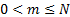) Domain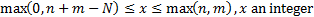Probability mass function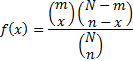Cumulative distribution function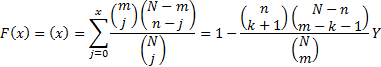where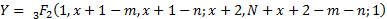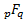is the generalised hypergeometric function, i.e.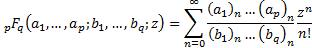and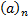involves the rising factorial or Pochhammer notation, i.e.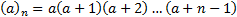and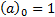Mean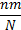Variance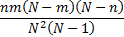Skewness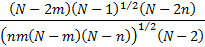(Excess) kurtosis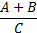where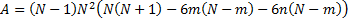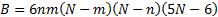Characteristic function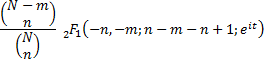Nematrian web functions

Functions relating to the above distribution may be accessed via the Nematrian web function library by using a DistributionName of “hypergeometric”. For details of other supported probability distributions see here.